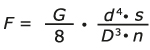# How do you calculate the force of a compression spring?

The force of a compression spring can be calculated using the following formula:There are many factors that affect the calculation of force. It is therefore recommended that we help you with calculations for your compression spring so you get the right result first time. We have calculation software that produces the right result, fast and accurately.

Fn (max. force) for stainless steel spring wire is approx. 0.88 x Fn for piano wire.

The calculation of spring force for a given length is travel s (in mm) x spring constant R (N/mm).

You are asked to state what material should be used based on the risk of corrosion, plus all the dimensions you want/have room for. In addition, any initial tension and travel should be stated. Based on this information, we can calculate what thickness of wire should be used, and how many coils the spring should have to provide the correct travel and force.

## Internet Explorer inkompatibilitet

Dette nettstedet fungerer dessverre ikke bra med Internet Explorer. Vi anbefaler å bruke en av følgende gratis nettlesere: